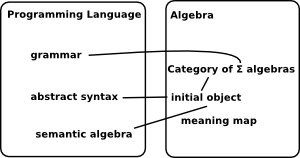# Semantics

Semantics aims to go beyond syntax in defining and proving the correct operation of a program. There are various ways we could theoretically construct a program from its elements:

• Operational Semantics - Defined in terms of the steps of the program execution.
• Axiomatic (Algebraic) Semantics - Defined in terms of axioms and rules.
• Denotional Semantics - Defined in terms of a mathematical model of the program.

We will look at these in more detail.

### Operational Semantics

We use logic to model a program and represent rules to rewrite it.

Commands in the language are modeled as taking some state to some other state. A notation for this could be:
(c,s) => t means the execution of command 'c' takes state 's' to state 't'.

We can then use logic to reason about such programs.

This concept of 'state' would include the values of the variables being considered. In logic terminology the context.

#### Big Step Semantics

Here we invent a minimal language to illustrate the how we could go about representing its semantics:

Language Element Syntax Description
Skip Skip Often in mathematics we need a way to represent no change such as '+ 0'
Assign Assign vname aexpr set a variable to the value of an expression
Seq Seq com com allow commands to be concatenated
If If bexpr com com conditional operation if then else
While While bexpr com loop

To implement the semantics we specify the logic as a set of rules:

 a noop (skip) can be added without changing meaning: | Skip (SKIP,s) => s a new assign can be added without changing meaning: | Assign (x := a,s) => s (x := aval a s) concatenation: (c1,s1) => s2 | (c2,s2) => s3 Seq (c1;c2, s1) => s3 bval b s | (c1, s ) => t IfTrue (IF b THEN c1 ELSE c2, s) => t ¬ bval b s | (c1, s ) => t IfFalse (IF b THEN c2 ELSE c1, s) => t bval b s1 | (c, s1) => s2 | (WHILE b DO c,s2) => s3 WhileTrue (WHILE b DO c,s1) => s3 ¬ bval b s WhileFalse (WHILE b DO c,s) => s

In operational semantics the 'meaning' of a program is defined in a formal way by defining how the program executes. We can then use logic proof principles such as derivation trees, rule inversion and rule induction.

 We can think of this as a diagram in some state space and so the blue arrows give equivalent way to get between these states.By defining a language in a formal way like this we may be able to prove things such as semantic equivalence between programs.

Parser: concrete syntax -> abstract syntax

### Denotational Semantics

We find some mathematical structure which we call a 'domain' to represent program phases. Typical domains are partial functions (poset).

It is important that we can compose these 'domains' so that program phases can be built out of 'subphrases'.

### Axiomatic (Algebraic) Semantics

In this case both syntactic and semantic entities have algebraic structure. Typically syntactic and semantic entities are expressed as elements of some algebra, and there is a morphism from syntax to semantics.

Any context-free language has the structure of an algebra of terms over a signature.

This approach seeks to study properties of programs subject to axiomatic assumptions about the range of possible semantic algebras.

 There is a connection between 'algebraic/axiomatic semantics' and category theory. Equations and initial algebras play a fundamental role in algebraic semantics.